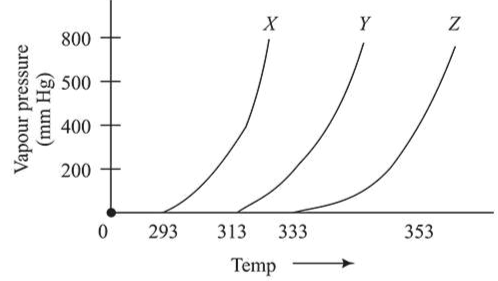# A graph of vapour pressure and temperature for three different liquids X, Y, and Z is shown below:Question:

A graph of vapour pressure and temperature for three different liquids $X, Y$, and $Z$ is shown below:(A) $X$ has higher intermolecular interactions compared to $\mathrm{Y}$.

(B) $X$ has lower intermolecular interactions compared to $\mathrm{Y}$.

(C) $Z$ has lower intermolecular interactions compared to Y.

The correct inference(s) is/are:

1. (A) and (C)

2. (A)

3. (B)

4. (C)

Correct Option: , 3

Solution:

At a particular temperature as intermolecular force of attraction increases vapour pressure decreases.

Thus, intermolecular forces are inversely proportional to vapour pressure and directly proportional to temperature.

Therefore X has lower intermolecular interactions compared to Y.